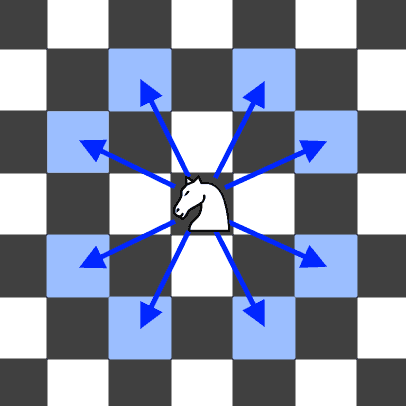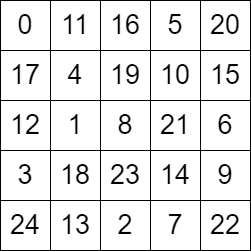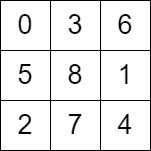##### Welcome to Subscribe On Youtube

Formatted question description: https://leetcode.ca/all/2596.html

# 2596. Check Knight Tour Configuration

## Description

There is a knight on an n x n chessboard. In a valid configuration, the knight starts at the top-left cell of the board and visits every cell on the board exactly once.

You are given an n x n integer matrix grid consisting of distinct integers from the range [0, n * n - 1] where grid[row][col] indicates that the cell (row, col) is the grid[row][col]th cell that the knight visited. The moves are 0-indexed.

Return true if grid represents a valid configuration of the knight's movements or false otherwise.

Note that a valid knight move consists of moving two squares vertically and one square horizontally, or two squares horizontally and one square vertically. The figure below illustrates all the possible eight moves of a knight from some cell.Example 1:Input: grid = [[0,11,16,5,20],[17,4,19,10,15],[12,1,8,21,6],[3,18,23,14,9],[24,13,2,7,22]]
Output: true
Explanation: The above diagram represents the grid. It can be shown that it is a valid configuration.


Example 2:Input: grid = [[0,3,6],[5,8,1],[2,7,4]]
Output: false
Explanation: The above diagram represents the grid. The 8th move of the knight is not valid considering its position after the 7th move.


Constraints:

• n == grid.length == grid[i].length
• 3 <= n <= 7
• 0 <= grid[row][col] < n * n
• All integers in grid are unique.

## Solutions

• class Solution {
public boolean checkValidGrid(int[][] grid) {
if (grid != 0) {
return false;
}
int n = grid.length;
int[][] pos = new int[n * n];
for (int i = 0; i < n; ++i) {
for (int j = 0; j < n; ++j) {
pos[grid[i][j]] = new int[] {i, j};
}
}
for (int i = 1; i < n * n; ++i) {
int[] p1 = pos[i - 1];
int[] p2 = pos[i];
int dx = Math.abs(p1 - p2);
int dy = Math.abs(p1 - p2);
boolean ok = (dx == 1 && dy == 2) || (dx == 2 && dy == 1);
if (!ok) {
return false;
}
}
return true;
}
}

• class Solution {
public:
bool checkValidGrid(vector<vector<int>>& grid) {
if (grid != 0) {
return false;
}
int n = grid.size();
vector<pair<int, int>> pos(n * n);
for (int i = 0; i < n; ++i) {
for (int j = 0; j < n; ++j) {
pos[grid[i][j]] = {i, j};
}
}
for (int i = 1; i < n * n; ++i) {
auto [x1, y1] = pos[i - 1];
auto [x2, y2] = pos[i];
int dx = abs(x1 - x2);
int dy = abs(y1 - y2);
bool ok = (dx == 1 && dy == 2) || (dx == 2 && dy == 1);
if (!ok) {
return false;
}
}
return true;
}
};

• class Solution:
def checkValidGrid(self, grid: List[List[int]]) -> bool:
if grid:
return False
n = len(grid)
pos = [None] * (n * n)
for i in range(n):
for j in range(n):
pos[grid[i][j]] = (i, j)
for (x1, y1), (x2, y2) in pairwise(pos):
dx, dy = abs(x1 - x2), abs(y1 - y2)
ok = (dx == 1 and dy == 2) or (dx == 2 and dy == 1)
if not ok:
return False
return True


• func checkValidGrid(grid [][]int) bool {
if grid != 0 {
return false
}
n := len(grid)
type pair struct{ x, y int }
pos := make([]pair, n*n)
for i, row := range grid {
for j, x := range row {
pos[x] = pair{i, j}
}
}
for i := 1; i < n*n; i++ {
p1, p2 := pos[i-1], pos[i]
dx := abs(p1.x - p2.x)
dy := abs(p1.y - p2.y)
ok := (dx == 2 && dy == 1) || (dx == 1 && dy == 2)
if !ok {
return false
}
}
return true
}

func abs(x int) int {
if x < 0 {
return -x
}
return x
}

• function checkValidGrid(grid: number[][]): boolean {
if (grid !== 0) {
return false;
}
const n = grid.length;
const pos = Array.from(new Array(n * n), () => new Array(2).fill(0));
for (let i = 0; i < n; ++i) {
for (let j = 0; j < n; ++j) {
pos[grid[i][j]] = [i, j];
}
}
for (let i = 1; i < n * n; ++i) {
const p1 = pos[i - 1];
const p2 = pos[i];
const dx = Math.abs(p1 - p2);
const dy = Math.abs(p1 - p2);
const ok = (dx === 1 && dy === 2) || (dx === 2 && dy === 1);
if (!ok) {
return false;
}
}
return true;
}